# Release notes#

## Version 1.4.0#

The following enhancements and bug fixes were implemented for this release:

### Fix y label for frequency response plots#

Fix the y label for the frequency response plots, showing ‘magnitude’ instead of displacement, velocity or acceleration.

### Documentation improvements#

Documentation improvements in the tutorials and class/functions missing from the API reference.

## Version 1.3.0#

The following enhancements and bug fixes were implemented for this release:

### Python 3.10 support#

This release adds python 3.10 support.

## Version 1.2.0#

The following enhancements and bug fixes were implemented for this release:

### Added Shape and Orbit classes#

We have added a `Shape` and an `Orbit` class to the `results` module. These classes are now responsible for calculating the mode shapes in the `ModalResults` class and also the deflected shapes in the `ForcedResponseResults` class. This simplifies the code since it avoids the repetition of code between these two classes. It also selects a single method for calculation of the major/minor axes and precession (forward or backward). Before, in the `ModalResults`, we were doing this using the method described by Friswell with the eigenvalues of the H matrix, and in the `ForcedResponseResults` we were using a calculation based on forward and backward vectors. The current code in the `Orbit` uses the method described by Friswell.

### Fix ucs map#

Fix calculation of bearing stiffness for plotting

Since we have removed the Coefficient class we no long calculate the bearing coefficient with bearing.kxx.interpolated. Now we use bearing.kxx_interpolated.

Fix number of modes

It was only possible to plot 4 modes. Now we can change the num_modes which is the number of calculated modes, and we will have that value divided by 4 being plotted. Value is divided by 4 because for each pair of eigenvalues calculated we have one wn, and we show only the forward mode in the plots, therefore we have num_modes / 2 / 2.

### Fix stiffness expression for tapered element#

In the stiffness expression described on appendix A of the Genta and Gugliotta paper, when substituting chi = 12EI/(phiGA*L**2) in the constant that multiplies the second matrix, we had canceled the A here with the Aj from the equation, but they are actually not the same area. The area Aj in the equation refers to the area in the element’s left side, while the area in the chi calculation refers to the area in the middle of the element. Therefore, we were having an error in this formula by a factor of Aj / A.

## Version 1.1.0#

The following enhancements and bug fixes were implemented for this release:

### Removed python 3.6 support#

Since we are now using pint 0.18 which requires python 3.7+, we can no longer support python 3.6.

### Add damping parameter and filtering to campbell#

• damping_parameter: choose between log_dec or damping_ratio;

• frequency_range: filter out modes that are out of this frequency range;

• damping_range: filter out modes that are out of this damping range;

### Improve bearing save method#

Save and load methods for bearings have been modified in order to have additional info in the save file, such as attributes used in bearing initialization.

### Change pkpk parameter used in plot#

The peak to peak parameter (pkpk) has been changed from prefixe to constant, which simplifies it use. To use peak to peak use ‘<unit> pkpk’ (e.g. ‘m pkpk’)

### Remove coefficient class#

Remove the auxiliary _Coefficient class in favor of a more simple data processing within the BearingElement class. The _Coefficient class was used to process the coefficient data, creating the interpolation functions and also providing plots (e.g. `bearing.kxx.plot()`). This was making the code more complicated since we basically had to reproduce the methods of an iterable object in this class. The discoverability of the plot function was also improved, since it is more natural for the user to search for a plot function within the bearing element and not as an attribute of each coefficient (`bearing.plot('kxx')` instead of `bearing.kxx.plot()` is now used). Another improvement regarding the plot is that the user can now pass multiple coefficients to plot with. Before the user would have to do something like:

```fig = bearing.kxx.plot()
fig = bearing.kyy.plot(fig=fig)
fig = bearing.kxy.plot(fig=fig)
fig = bearing.kyx.plot(fig=fig)
```

Now we can do:

```bearing.plot(coefficients=['kxx', 'kyy', 'kxy', 'kyx'])
```

### Correct an error in the damping coefficient of the Magnetic Bearings Element#

An small error in the magnetic bearing calculation has been corrected. See #816.

### Fix error in static analysis for bearings with high stiffness#

This adds an auxiliary rotor with 0 stiffness in the bearing location to fix calculations, otherwise, a rotor with a bearing with high stiffness would have 0 reaction force at the bearing location.

### Fix error in static analysis for bearings and disks added to the same node#

For rotors with a bearing and a disk added to the same node the static analysis would fail. See #845 for more details.

### Add check units to run modal#

Run modal was not working with units. Now we can use rotor.run_modal(speed=Q_(1000, “RPM”).

## Version 1.0.0#

The following enhancements and bug fixes were implemented for this release:

### Add loop to calculate frequency-depedent coefficients in from_fluid_flow()#

Users will be able to input as many frequency values as needed and `from_fluid_flow()` will run a loop calculating a set of coefficients for each given frequency. Now, `omega` argument must be a list of frequencies. It also changes the main function used to calculate the coefficients. `calculate_stiffness_and_damping_coefficients()` is now used to do it numerically.

### Extract fluid flow#

The .from_fluid_flow method has been extracted from the BearingElement to a class called BearingFluidFlow. This is more in line with how we have all the Bearing classes, and makes it easier for the user to find it.

### Add minor and major axes to response plots#

We can now select the major and minor axis when plotting responses:

```   # plot response for major or minor axis:
>>> probe_node = 3
>>> probe_angle = "major"   # for major axis
>>> # probe_angle = "minor" # for minor axis
>>> probe_tag = "my_probe"  # optional
>>> fig = response.plot(probe=[(probe_node, probe_angle, probe_tag)])

Calculate velocity and acceleration responses
```

The method `run_freq_response()` now returns, not only the displacement results, but also the velocity and acceleration. The data is then input to `FrequecyResponseResults` and `ForcedResponseResults` classes.

The user is able to choose the response by changing the amplitude_units argument.
• Inputing ‘[length]/[force]’, it displays the displacement;

• Inputing ‘[speed]/[force]’, it displays the velocity;

• Inputing ‘[acceleration]/[force]’, it displays the acceleration.

### Add units to stochastic ross module#

Units use is now possible in the stochastic module.

### Crack, rubbing and misalignment simulation#

It is now possible to run simulations for some defects such as cracks, rubbing and misalingment.

### Change methods to run_#

The plot_level1 and plot_ucs methods were refactore so that they behave as other run methods. Now we the run* prefix to call them and they will return Results objects.

```ucs = rotor.run_ucs(...)
ucs.plot(...)
```

### Fix GlobalIndex with non integer values#

`PointMass` and `BearingElements` with `n_link` argument were getting non integer values. Matrices would not be built, once these values are used as reference to array indexes (which must be integers).

## Version 0.4.0#

The following enhancements and bug fixes were implemented for this release:

### Class MagneticBearingElement was created#

This class creates a magnetic bearing element. The element can be created by:

• defining directly the stifness and damping coefficients with the method **init**;

• defining the magnetic parameters g0, i0, ag, nw, alpha and the porportional and derivative gains of a PID controller kp_pid, kd_pid with the method param_to_coef.

The reference for the equations is: Book: Magnetic Bearings. Theory, Design, and Application to Rotating Machinery Authors: Gerhard Schweitzer and Eric H. Maslen Page: 84-95 (magnetic parameters) and 354 (PID gains)

### Stochastic Ross module#

The files follows the hierarchy than ROSS: different files to build the elements and a main file to build the rotors.

The main difference between these new files and and the element files from ROSS is that the stochastic elements files build list of elements instead a single one.

The analysis will also follow the ROSS structure, but it’s not added yet.

### 6dof assembly#

Generalization of the assembly and evaluation codes of ROSS for a generic “N” DoF sized formulation. This is intended to enable the 6 DoF implementation to be executed appropriately, and is made in a generalized fashion by default.

### Add scale_factor argument to DiskElement class#

Argument to scale the disk patch. Used to increase (scale_factor > 1.0) or reduce (scale_factor < 1.0) the size of disk patches when plotting the rotor.

### Add units to the DiskElement, BearingElement and PointMass classes#

Arguments for these classes can be passed as pint.Quantity now.

### Replacing plotting libraries#

We have replaced Matplotlib and Bokeh plotting libraries with Plotly.

Add methods to plot the deflected shape

### Move “sparse” argument to run_modal()#

The eigenvalue / eigenvector solver is chosen when running the run_modal() method, and not anymore an parameter from the rotor model

### Set default values to log_dec color scale#

The color scale to represent the log_dec values ranges between 0 and 1, independently of the log_dec values calculated. It can be manually modified by insert 2 simple kwargs: coloraxis_cmin and coloraxis_cmax. It will change respectively the minimum and maximum values for the color scale. Also adds exponentformat = “none” to X and Y axis for better visualization of values

Example with default options:

```>>> speed_range = np.linspace(0, 3000, 51)
>>> campbell = rotor.run_campbell(speed_range)
>>> campbell.plot(harmonics=[0.5, 1])
```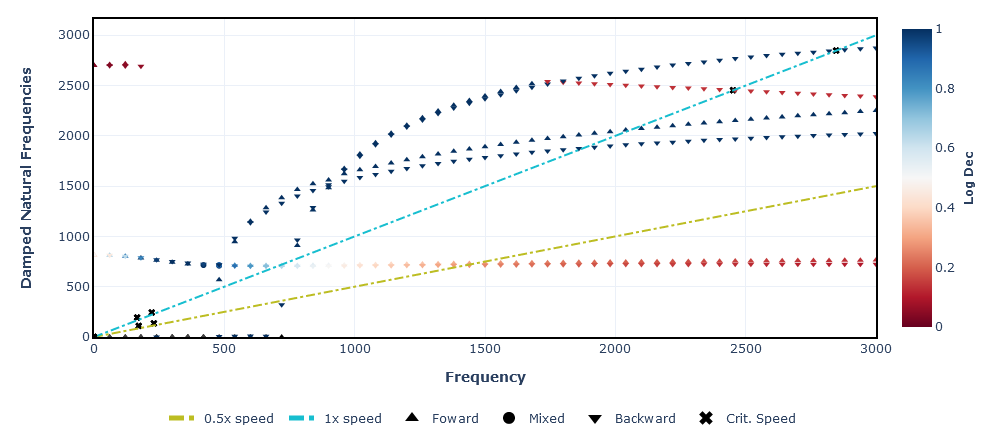Example changing the max value for the scale:

```>>> speed_range = np.linspace(0, 3000, 51)
>>> campbell = rotor.run_campbell(speed_range)
>>> campbell.plot(harmonics=[0.5, 1], coloraxis_cmax=2.0)
```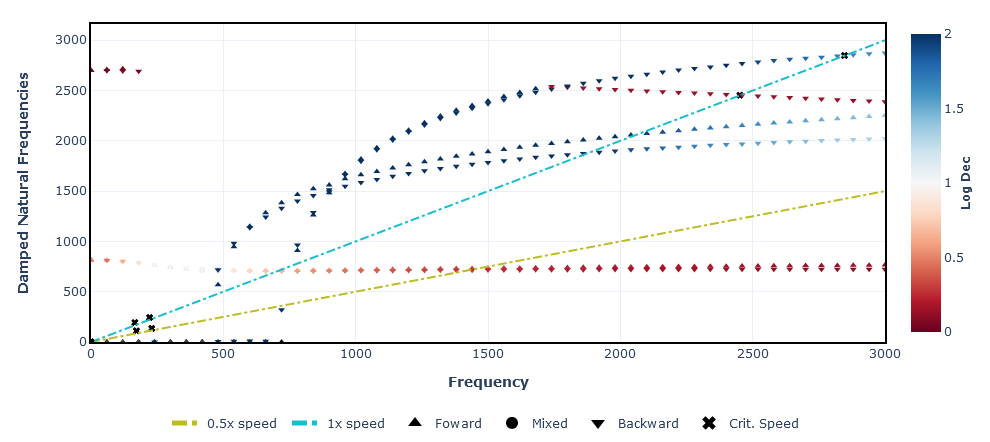### Replace interp1d by line_shape from Plotly#

It removes the need of creating sub arrays from interpolation to smooth some results in StaticResults.

### Add probe argument to ForcedResponseResults#

Replace `dof` by `probe`argument probe is a list of tuples. Each tuple refers to a probe, which must include de node and the orientation.

The orientation units is set by `probe_units` argument. in `plot()` method, `data.showlegend` is turn off to avoid repetitive legend marks on graphs

We have change most methods to use the word ‘save’ and ‘load’ instead of ‘get’, ‘use’ etc.

### Move “sparse” argument to run_modal()#

The eigenvalue / eigenvector solver is chosen when running the run_modal() method, and not anymore an parameter from the rotor model

### Fix issue with check_units#

Related to Issue #511

Add a condition to verify if the argument value is None. It allows the user to pass “None” args without getting an error with the code trying to assign a unit to a None value.

### Fix missing parameters in ST_ShaftElement#

• The parameters `axial_force` and `torque` were missing from the `ST_ShaftElement.__init__()`.

### Fix missing y_pos and y_pos_sup in df_seals#

Related to Issue #571.

Seals can be displayed in different colors than bearings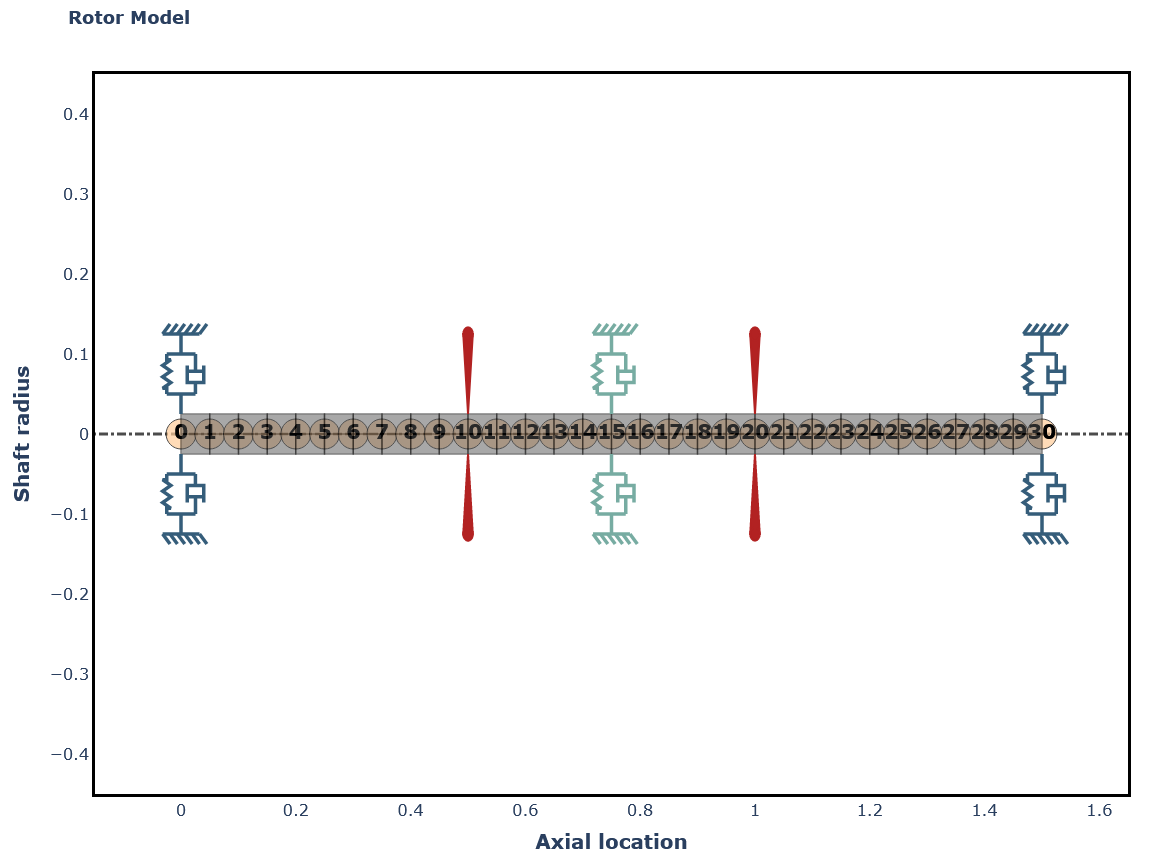### Fix ValueError when inputting an integer to disk properties#

Related to Issue #593.

## Version 0.3.0#

The following enhancements and bug fixes were implemented for this release:

### Remove ShaftElement and replace with the ShaftTaperedElement#

Now we only have one class for the shaft element which is capable of handling cylindrical and tapered elements.

### Allow user choose material in “Rotor.from_section()”#

As requested in Issue #401, the user will be able to define a material when using `.from_section()` classmethod. In addition, more modifications have been done regarding the new ShaftElement API. Now, the user has the option to instantiate tapered sections.

### CoAxialRotor class#

A class is now available for modeling coaxial rotors.

### Fix bearing position bug#

Fix bug mentioned in Issue #276

Sorting the bearing_seal_elements make it to cycle correctly while assuming `y_pos` values to them.

## Version 0.2.0 (beta release)#

The following enhancements and bug fixes were implemented for this release:

### Improvements for plotting results#

This PR make some modifications in results.py

• Add show(camp) to show campbell bokeh plotting

• Add command to allign ColorBar axis for better looking

• Add figure dimensions for campbell bokeh plotting

• increases the font size of all axis labels

Some bokeh figures have the attribute `sizing_mode="stretch_both`. This command line is used so that the plotting of the graphics is always the maximum size of the browse. However, this creates a bug in jupyter notebook, and the graphs does not appears. So it should be replaced by `width` and `height` command lines

• adds an interpolation function to highlight to the user the intersection between the critical frequency curves and the speed line

### Index method#

Adds `.dof_local_index()` and `.dof_global_index()` methods to the elements. These methods will return namedtuples with the index for each coordinate based on the following convention:As an example:

```>>> import ross as rs
>>> from ross.materials import steel

>>> le = 0.25
>>> i_d = 0
>>> o_d = 0.05
>>> sh_el = rs.ShaftElement(le, i_d, o_d, steel, n=2)
>>> sh_el.dof_local_coordinates()
LocalIndex(x0=0, y0=1, alpha0=2, beta0=3, x1=4, y1=5, alpha1=6, beta1=7)
>>> sh_el.dof_global_coordinates()
GlobalIndex(x0=8, y0=9, alpha0=10, beta0=11, x1=12, y1=13, alpha1=14, beta1=15)
```

With this we can change the following code:

```for elm in self.shaft_elements:
n1, n2 = self._dofs(elm)
M0[n1:n2, n1:n2] += elm.M()
for elm in self.disk_elements:
n1, n2 = self._dofs(elm)
M0[n1:n2, n1:n2] += elm.M()
```

To:

```for elm in self.elements:
dofs = elm.dof_global_index()
n0 = dofs
n1 = dofs[-1] + 1  # +1 to include this dof in the slice
M0[n0:n1, n0:n1] += elm.M()
```

### interactions with rotor plotting#

When plotting a rotor, the user will be able to check some parameters of the elements in the output image itself, just by moving the cursor over the desired element, for example:

By moving the cursor over a shaft element the user will see this: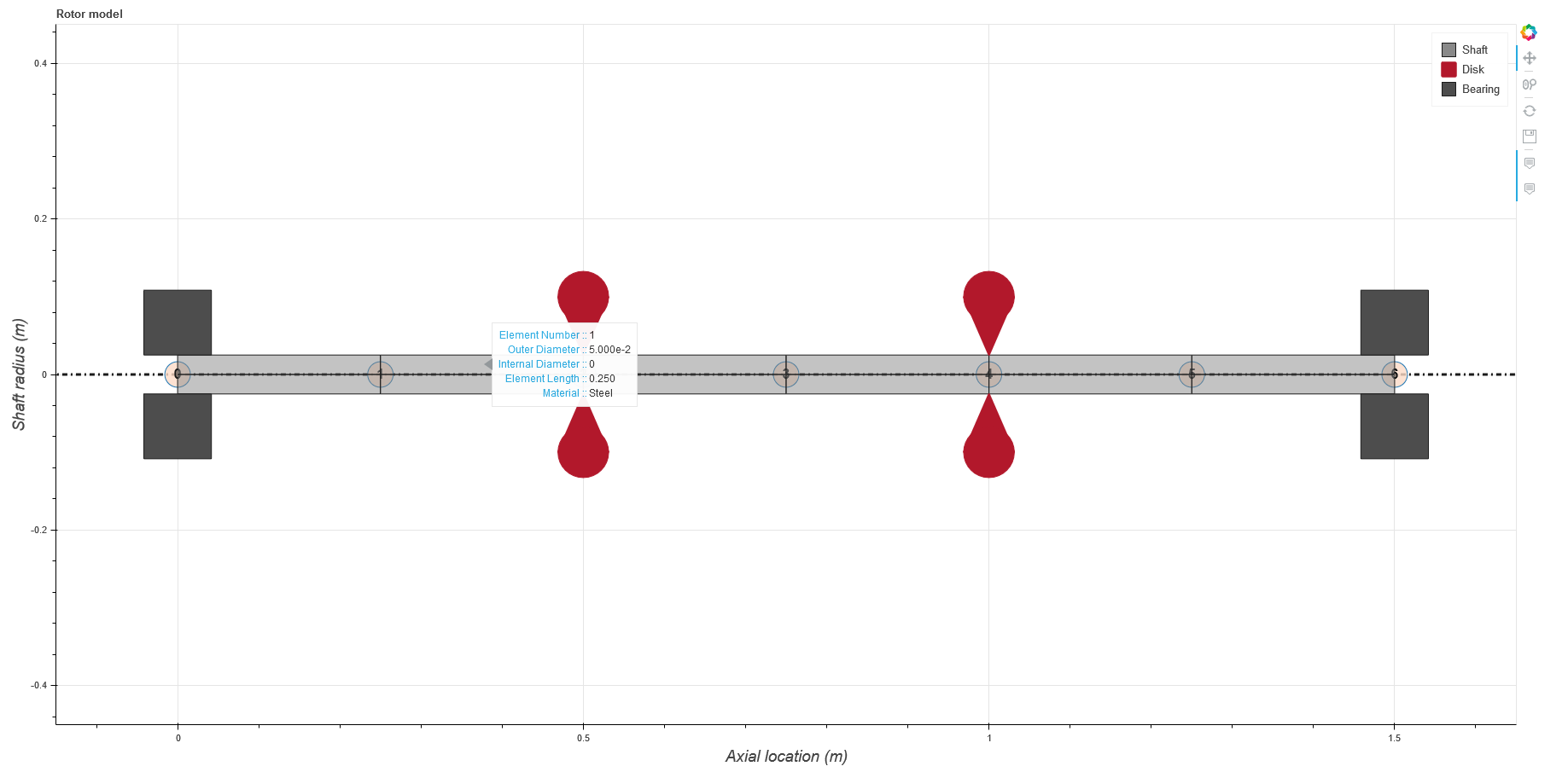And, by moving the cursor over a disk element the user will see this: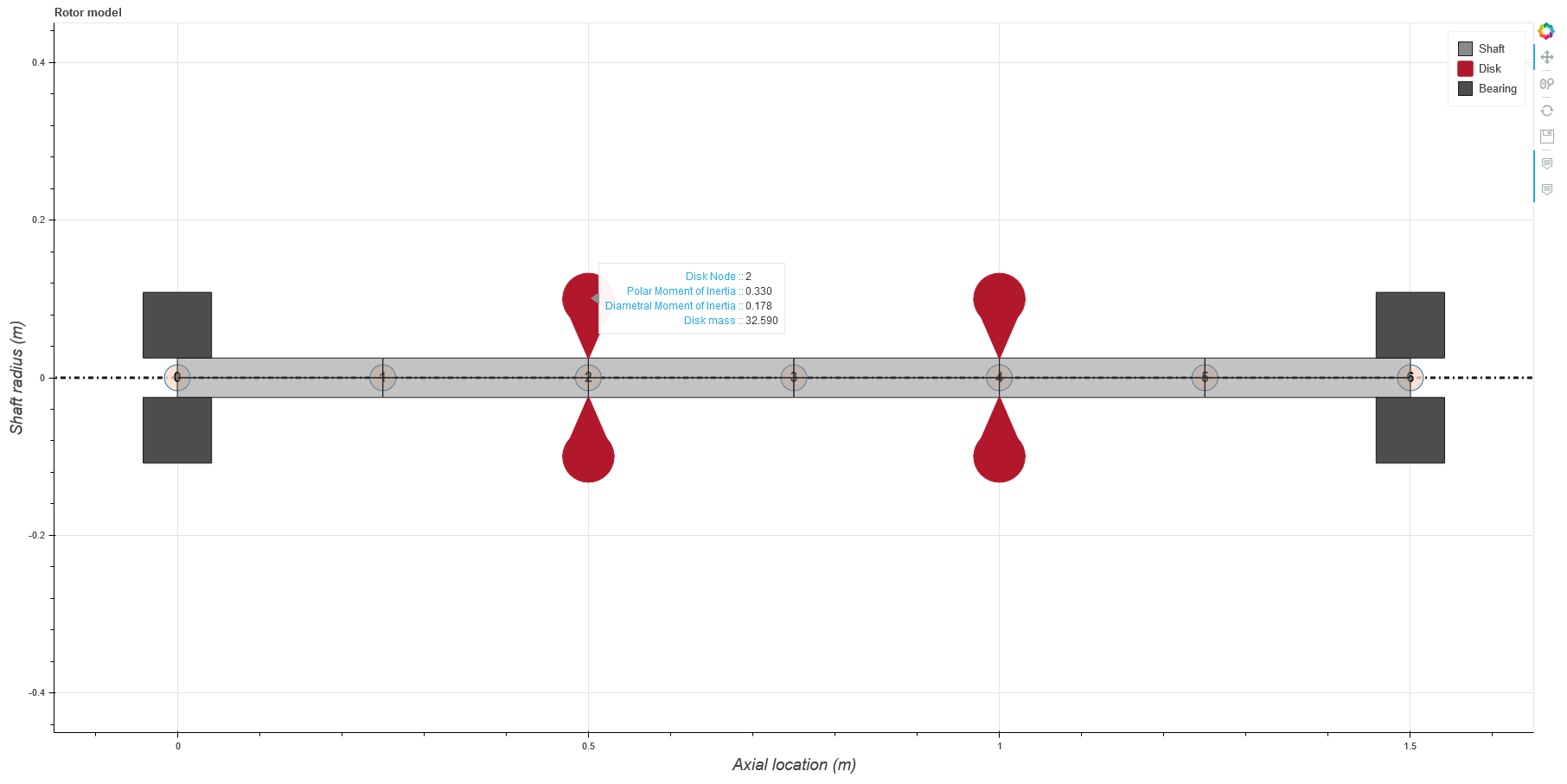I didn’t add the same functionality to bearing elements because the coefficients may vary with rotor speed, and this may cause a misunderstanding to the user.

### Move time response plotting to results#

• Removes the code related to plotting from this file. It was transfered to results.py

• Replaces the method name from plot_time_response to run_time_response.

• Fix ForcedResponseResults docstring

• Add new element: tapered shaft element

• This class have, basically, the same inputs than “ShaftElement”. The difference relies on entering the diameters of each side of the element (left and right).

• Patches methods were adapted to draw the conical shape of the element.

The matrices generated for this element follow the reference below.

Genta, G., and Gugliotta, A. (1988). A conical element for finite element rotor dynamics, Journal of Sound and Vibration 120,175-182.

### Improving plot rotor#

This PR modifies the plotting size of disks, bearings and seals. The size, previously based on shaft length, is now calculated based on the shaft diameter.

### Add Check slenderness ratio method#

Adds a method that will return the colored rotor plot if the condition to the mininum slenderness ratio is not met.

Now using `.plot.rotor()` won’t display the colored rotor anymore. Instead, the user will call `.check_slenderness_ratio()` to check this attribute.

### Make Free-Body Diagram more readable#

Pull Request to fix Issue #243

• Slightly increases the size of the figures

• Arrows have a fixed length now (as shown in this figure below);

• Arrows now have different colors to distinguish forces (from shaft, disks or bearings/seals);

• Labels were rotated 90º;

• Text font size reduced to “9pt”;

• Y axis is hidden. The force values are only displayed only next to the arrows, without size ratio.

### Replace bearing plot style / remove element length dependency from glyphs#

• Bearing representation changes from a simple square to a spring-damper set.

• Fix bug glyphs were plotted on the minimum outer diameters of a shaft node.

• Removes length attribute from `patch()` and `bokeh_patch()` of BearingElement and DiskElement classes.

Bokeh plot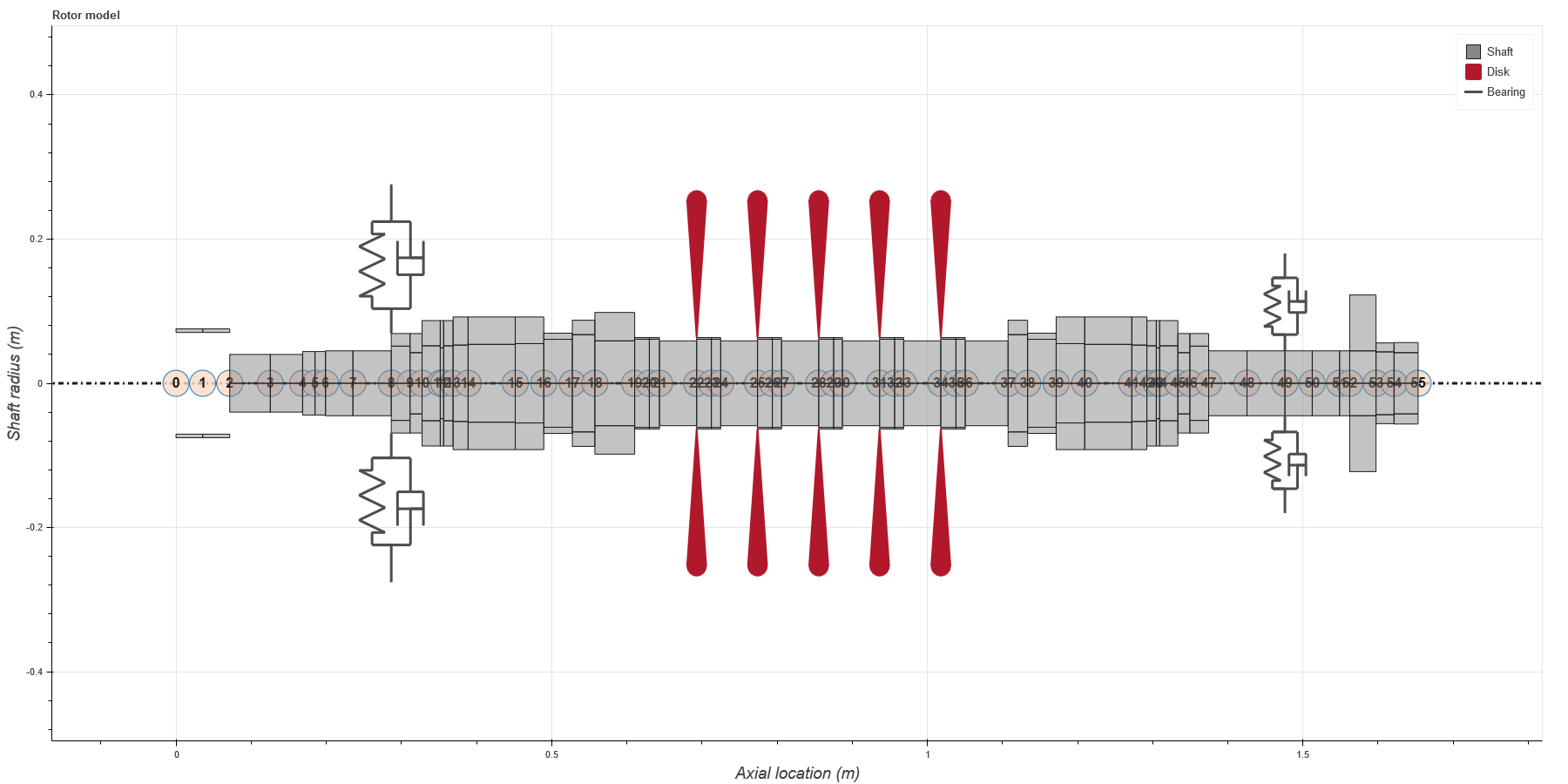Matplotlib plot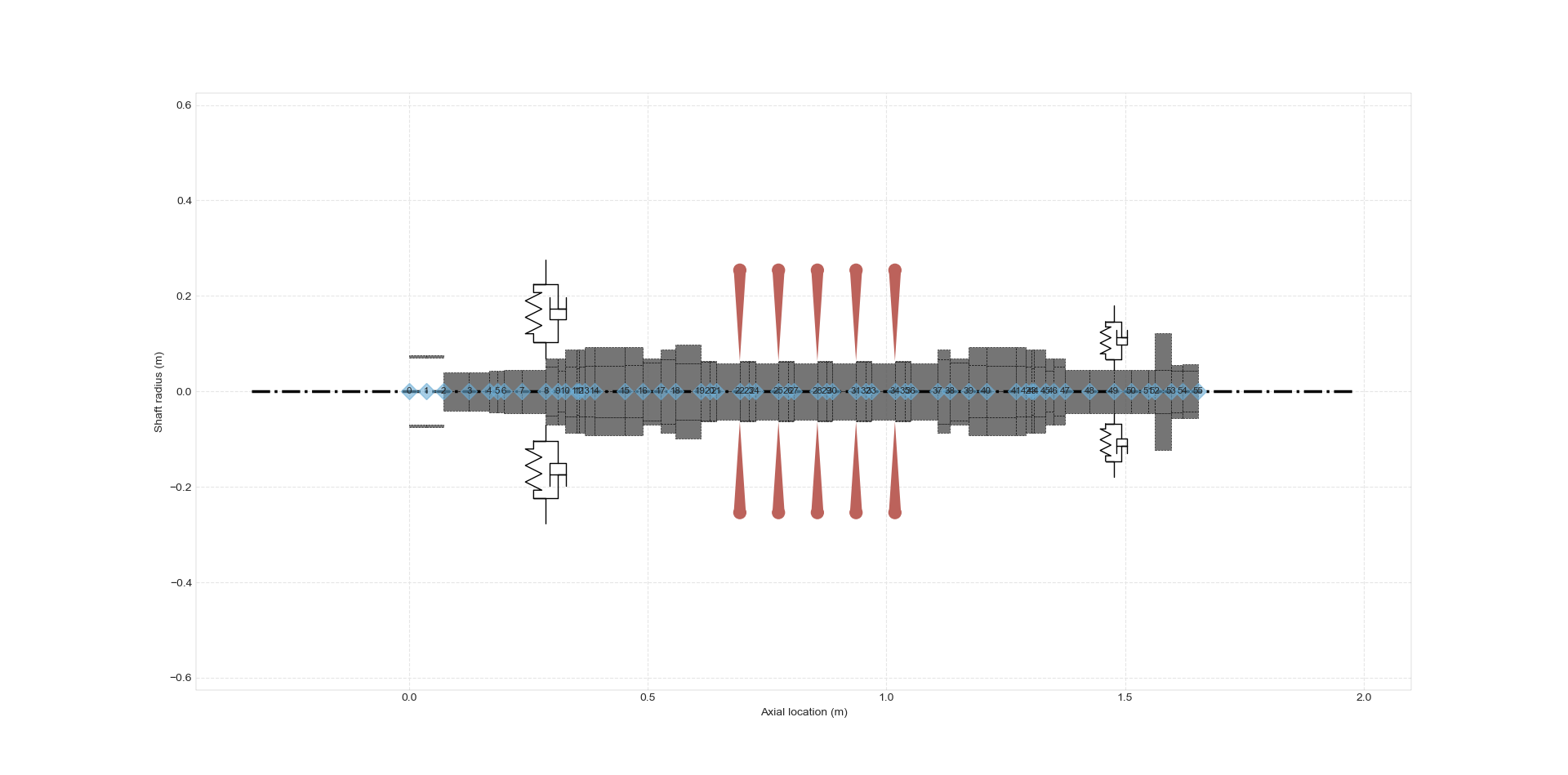Work in Progress

This PR adds some plotting styles follwing the API reference:

• Separation Margin

• Amplification Factor

• Table of API parameters

It follows the Issue #195

### Add new “Tag” attribute to elements#

Disk, bearing and seal elements now get an attribute called “Tag”. This allows the user to name the elements to help refer to the actual equipment (impellers, blades, labyrinth seal…)

If the user do not input a tag, it’s named after:

• Disk 1, 2, 3…

• Bearing 1, 2, 3…

• Seal 1, 2, 3…

As bearings and seals are input in the same list of objects, this situation below may happens:

```def rotor_example():
i_d = 0
o_d = 0.05
n = 6
L = [0.25 for _ in range(n)]

shaft_elem = [
ShaftElement(
l, i_d, o_d, steel, shear_effects=True, rotary_inertia=True, gyroscopic=True
)
for l in L
]

disk0 = DiskElement.from_geometry(
n=1, material=steel, width=0.07, i_d=0.05, o_d=0.28
)
disk1 = DiskElement.from_geometry(
n=3, material=steel, width=0.07, i_d=0.05, o_d=0.28, tag="disk_test"
)
disk2 = DiskElement.from_geometry(
n=5, material=steel, width=0.07, i_d=0.05, o_d=0.28, tag="disk_test2"
)

stfx = 1e8
stfy = 1e8
bearing0 = BearingElement(0, kxx=stfx, kyy=stfy, cxx=1000, tag="brg")
bearing1 = BearingElement(6, kxx=stfx, kyy=stfy, cxx=1000)
seal0 = SealElement(2, kxx=1e2, kyy=1e2, cxx=500, cyy=500, tag="seal")
seal1 = SealElement(4, kxx=1e2, kyy=1e2, cxx=500, cyy=500)

return Rotor(shaft_elem, [disk0, disk1, disk2], [bearing0, seal0, seal1, bearing1])

>>> rotor = rotor_example()
>>> rotor.df_bearings["tag"]
0    brg
1    seal
2    Seal 2
3    Bearing 3
Name: tag, dtype: object
```

The counting for bearings and seals is not independent. I don’t see it as big deal, but I’m open to suggestions.

### Add separated DataFrame to seals#

This splits bearings from seals in two different dataframes:

• df_bearings for bearings

• df_seals for seals

It aims to better organize the elements and to avoid code interpretation problems such as in static analysis:

This is the free-body diagram of an example while seals and bearings are merged in same dataframe. The code interprets seals like bearings and calculate reaction forces where both seals are placed: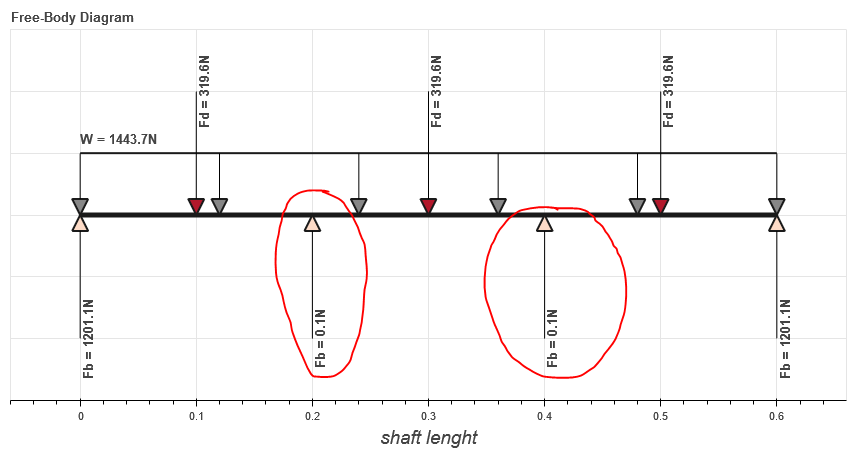And now, spliting bearings and seals: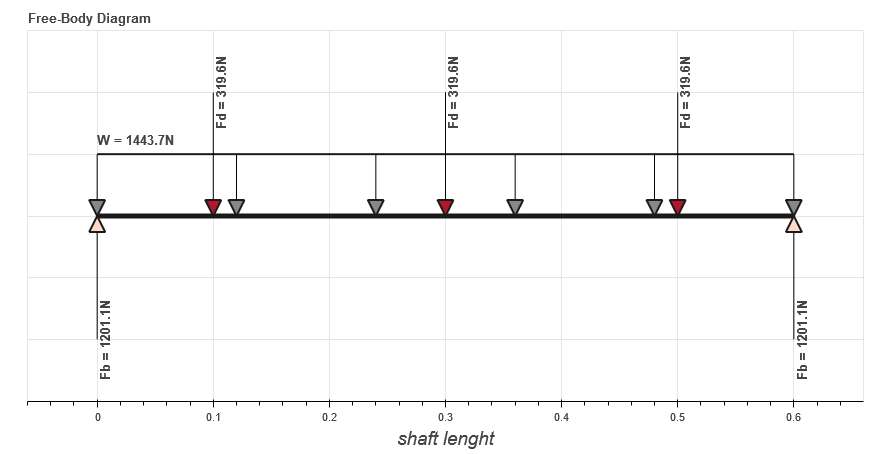Also, fix a bug when modeling too flexible bearings, displacement were getting larger than acceptable. It uses axuliar bearings with high stiffness, considering almost zero displacement in bearing nodes.

### Split StaticResults plot method#

This commit splits the plot method in four:

• plot_deformation()

• plot free_body_diagram()

• plot_shearing_force()

• plot_bending_moment() This is related to Issue #303.

### Add BallBearing and RollerBearing Element#

This PR adds two new classes:

• BallBearingElement

• RollerBearingElement

These classes create a bearing element for ball and roller bearings models. They are instantiated based os some geometric and constructive parameters like: number of rotating elements, size (diameter for ballbearing; length for rollerbearing) of the rotating elements, contact angle, and static loading force.

The direct stiffness coefficientes are calculated using these parameters.

I also left an opened option to instantiate the direct damping coefficients. But if it’s set as None, they are calculated based on the stiffness coefficient as the literature suggests. However the cross-coupling coefficients are set to zero, and the coefficients are not dependent on speed.

```def __init__(
self,
n,
n_balls,
d_balls,
fs,
alpha,
cxx=None,
cyy=None,
tag=None,
):

Kb = 13.0e6
kyy = (
Kb * n_balls ** (2./3) * d_balls ** (1./3) *
fs ** (1./3) * (np.cos(alpha)) ** (5./3)
)

nb = [8, 12, 16]
ratio = [0.46, 0.64, 0.73]
dict_ratio = dict(zip(nb, ratio))

if n_balls in dict_ratio.keys():
kxx = dict_ratio[n_balls] * kyy
else:
kxx = f(n_balls)

if cxx is None:
cxx = 1.25e-5 * kxx
if cyy is None:
cyy = 1.25e-5 * kyy

super().__init__(
n=n,
w=None,
kxx=kxx,
kxy=0.0,
kyx=0.0,
kyy=kyy,
cxx=cxx,
cxy=0.0,
cyx=0.0,
cyy=cyy,
tag=tag,
)
```

### Add condition to ShaftTaperedElement instantiation#

• Add a condition if the user do not attribute any value to inner and outer diameters on the right side, the values are automatically set to be equal the left side.

• Also, move the “material” input to the first position, so the geometric parameters can be grouped together.

• Adapt the examples in docstring.

• Moves the inputs from ShaftTaperedElement to match the new position set.

```class ShaftTaperedElement(Element):
def __init__(
self,
material,
L,
i_d_l,
o_d_l,
i_d_r=None,
o_d_r=None,
n=None,
axial_force=0,
torque=0,
shear_effects=True,
rotary_inertia=True,
gyroscopic=True,
shear_method_calc="cowper",
tag=None,
):

if i_d_r is None:
i_d_r = i_d_l
if o_d_r is None:
o_d_r = o_d_l
```

### Patches for PointMass element#

As required by issue #310

### Add __repr__, __eq__, and example() to point mass element#

This commit adds the following methods to PointMass class:

• repr - representative method;

• eq - comparasion method;

• point_mass_example() - to run some doctests.

### Improvements to Static Analysis Documentation#

• Related to Issue #327

• Remove force_data dictionary

• Get the items and transform them in Rotor attributes

• shaft_weight - Shaft total weight

• disk_forces - Weight forces of each disk

• bearing_reaction_forces - The static reaction forces on each bearing

### General Modifications in CampbellResults#

This is related to Issues #326 and #328.

• Introduce a condition to add the hover tool only if an harmonic crosses a critical speed curve.

• Remove some unused imports.

• In _plot_bokeh:

• Change colormap from viridis to Red-Blue

• Add diferent colors to harmonics lines

• Make glyphs on legend with same color

• In _plot_matplotlib:

• Add diferent colors to harmonics lines

• For both methods: Restructure code to increase efficiency (reduce plotting time): I could the plot time in an half by rearranging some routines.

```import ross as rs
rotor = rs.rotor_example()
camp = rotor.run_campbell(np.linspace(0, 1000, 10)).plot([0.25, 0.5, 1, 2])
show(camp)
```

I’ve measured the time just to run the .plot(). It was taking 2.5s for the rotor_example(). Rearranging the code, it has been reduced to 1.2s for this same example.

### Add summary table to plot rotor information#

Related to Issue #327.

It’s a first idea from what the summary will become. The class get the values stored in dataframes and turns them into a bokeh widget in table format.

We still can build other tables to make it more complete and useful to manipulate data.

### Improvements to SummaryResults#

Related to Issue #327 Follows the PR #338

• Add new attribute “tag” to name the rotor;

• Add CG and Moment of Inertia parameters;

• Add new attributes to .summary() method;

• Add a system of Tabs that allows the user to alternate between tables;

• Tables are separeted in:

• Rotor summary

• Shaft summary

• Disk summary

### Add Stability Level 1 analysis#

• This analysis consider a anticipated cross coupling QA based on conditions at the normal operating point and the cross-coupling required to produce a zero log decrement, Q0.

• This attribute is necessary to distinguish overhung and between bearings rotors.

• The unbalance forces and and the respective nodes where they are introduced depends on this information.

• Improve unbalance force method.

• With this improvement, the unbalance forces are calculated according to the rotor_type and consideres the disks and shaft wheight (if overhung).

• Change mode_shape to find nodes according to rotor_type

• The method .mode_shapes() was capturing the maximum points from the rotor’s mode shapes without checking if it was overhung or between bearings.

• For rotors where the disk is cantilevered beyond the bearings, unbalance shall be added at the disk. So, the method now checks the rotor_type to define the nodes correctly.

• Add machine_type and disk_nodes attribute

• Machine_type will be useful to help the code to distinguish between compressors, turbines, etc. Because each machine type has their own conditions to be calculated.

• Disk_nodes is useful to collect the disk of interest. if we are working with a overhung rotor, disk_nodes will collect the disk which are overhung only, for example.

This Level 1 analysis does not have the screening criteria yet. The next step is to implement the Level 2 analysis. Once I get to work on it, I’ll add the screening criteria. I still need to organize some ideas for the next step.

• add a summary table for bearing elements: for now it displays only where it’s placed and the reaction forces.

• fix bug: when setting and disk or bearing element in the last node, the code would fail

• other minor changes

### Improvements to api_report.py - Add test_api_report.py#

• Add Stability level 1 screening criteria;

• Modifies the code to storage the cross-coupling range for each rotor stage (or impeller). This is useful to distinguish the cross-coupling evaluation for different `rotor_types` (between bearings, overhung…);

• Add `.summary()` method;

• Add summary for stability level 1

### Add stability analysis level 2#

For the level 2 stability analysis additional sources that contribute to the rotor stability shall be considered. These sources shall replace the cross-coupling Qa, calculated in the stability analysis level 1

### Visual improvements to graphs#

The labels, ticks and titles font size from bokeh figure were small when putting it presentations. So I’ve increased the font size of all graphs.

• Add new tab to the `.summary()` with stability level 2 data;

• Change stability level 2 labels for better user understanding when using `.summary()`. The labels include explicitly all the components considered in a certain analysis (Shaft + Bearing + Disk + Seal…);

• Increases labels, titiles and tick font sizes (results.py and api_report.py);

• Add mcs speed to evaluate mode shapes (api_report.py);

• Fix `.unbalance_response()` plot size to match results.py file;

• In `.stability_level_1()` remove condition from returning and add it as attribute;

• `.run_modal().plot_mode()` add legend informing the whirl direction.

### Add method to plot orbit#

• Add `.run_orbit_response()` method to rotor_assembly.py.

• `.run_orbit_response()` calculates the orbit for a rotor’s given node, speed and forces.

• Add class `OrbitResponseResults` to results.py.

• Class used to store results and provide plots for orbit response.

Example using this new method:

```>>> rotor = rotor_example()
>>> speed = 500.0                                           # pick a rotor speed
>>> size = 10000                                            # time array's size
>>> node = 3                                                # node of interest
>>> t = np.linspace(0, 10, size)                            # create time array
>>> F = np.zeros((size, rotor.ndof))                        # create force vectors
>>> F[:, 4 * node] = 10 * np.cos(2 * t)                     # introduce a periodic force in a single dof
>>> F[:, 4 * node + 1] = 10 * np.sin(2 * t)                 # introduce a periodic force in a single dof
>>> response = rotor.run_orbit_response(speed, F, t, node)  # run orbit response
>>> show(response.plot())                                   # plot orbit response
```

### 3D plots for orbit response#

Related to PR #385.

#### Bug fixes#

Fix Campbell’s strange behavior for precession

### Fix equality for bearing element#

Fixes the equality for bearing element, allowing the comparison with objects from different types, e.g. bearing == 1 will return False, before an AttributeError was raised since int doesn’t have .dict. This also removes pytest as a dependence for the user.

The import has been added to the results module because the mode shape plot needs this to work. Although it looks like the import is not being used (this is highlighted by linters), matplotlib, for some strange reason, actually needs this to use the projection=’3d’. A doctest has been added to the mode shape plot for the tests to fail if this import is removed.

### Fix bearing type definition and add new test#

Fix the bearing type definition and also add a new test that checks if a second analytical way to calculate the pressure matrix matches the numerical way. Close #175

### Improvements for plotting results#

This PR make some modifications in results.py

• Add show(camp) to show campbell bokeh plotting

• Add command to allign ColorBar axis for better looking

• Add figure dimensions for campbell bokeh plotting

• increases the font size of all axis labels

Some bokeh figures have the attribute `sizing_mode="stretch_both`. This command line is used so that the plotting of the graphics is always the maximum size of the browse. However, this creates a bug in jupyter notebook, and the graphs does not appears. So it should be replaced by `width` and `height` command lines

• adds an interpolation function to highlight to the user the intersection between the critical frequency curves and the speed line

### Fix bug - plotting unbalance response#

Unbalance response was not plotting when calling it using matplotlib

### Make Free-Body Diagram more readable#

Pull Request to fix Issue #243

• Slightly increases the size of the figures

• Arrows have a fixed length now (as shown in this figure below);

• Arrows now have different colors to distinguish forces (from shaft, disks or bearings/seals);

• Labels were rotated 90º;

• Text font size reduced to “9pt”;

• Y axis is hidden. The force values are only displayed only next to the arrows, without size ratio.

Fix inconsistencies in the mass and gyroscopic matrices; New tests for shaft tapered element

I found some problems in shaft tapered element matrices. Fow now I’ve fixed the issues on mass and gyroscopic matrices. Besides this PR modifies the tests for this class, adding tests to compare two cylindrical elements built from `ShaftElement` and `ShaftTaperedElement` classes. However, tests for stiffness matrices are skipped.

### Fix typo in __repr__#

It follows the Issue #255.

### Fix inconsistencies in the stiffness matrix#

• Fix some typos in geometry coefficients;

• Fix stiffness matrix formula for shaft tapered elements;

• Fix docstrings for M(), K(), G() in ShaftTaperedElement;

• Add better docstrings to ShaftTaperedElement.

Fix tests to match the changes done in shaft_element.py:

• Fix stiffness matrix test;

• Fix element attribute tests ;

• Fix comparation tests.

### Fixing doctests#

Well, since I have forgotten to add the .py extention on api_report file, the CI’s were not running the tests on it. And now that I did it, the building is failing due some tests.

This PR tries to fix this problem on api_report.py and in results.py (with new docstrings on ModeShapeResults)

The last PR (#272) was a failure actually.

### Adapt convergence analysis to ShaftTaperedElement#

Now the convergence analysis uses the class ShaftTaperedElement to generate shaft elements since it’s more generic. This should solve issue #280.

### Add separated DataFrame to seals#

This splits bearings from seals in two different dataframes:

• df_bearings for bearings

• df_seals for seals

It aims to better organize the elements and to avoid code interpretation problems such as in static analysis:

This is the free-body diagram of an example while seals and bearings are merged in same dataframe. The code interprets seals like bearings and calculate reaction forces where both seals are placed:And now, spliting bearings and seals:Also, fix a bug when modeling too flexible bearings, displacement were getting larger than acceptable. It uses axuliar bearings with high stiffness, considering almost zero displacement in bearing nodes.

### Fix warning when changing number of nodes to be plotted#

Related to Issue #295

### Remove unit conversion factor#

Remove a conversion factor (meters to milimeters) from the plot_deformation() ColumnDataSource. So the x and y axis units get to be the same.

Related to Issue #312

### Improvements to Static Analysis Documentation#

• Related to Issue #327

• Remove force_data dictionary

• Get the items and transform them in Rotor attributes

• shaft_weight - Shaft total weight

• disk_forces - Weight forces of each disk

• bearing_reaction_forces - The static reaction forces on each bearing

### Fix bearings not connecting to tapered elements#

Related to Issue #332

### Fix bug when plotting bearings#

• Fix bug when plotting bearing elements in a given node with more than 1 shaft element on it. The bearing would not get correct starting point.

• Fix bug when using scale_factor with point mass. The auxiliar bearing would disattach from the first bearing.

### Fix bug in .run_freq_response()#

Fix a bug that happens when calling `.run_freq_response()` with default arguments, i.e:

```>>> rotor = rotor_example()
>>> response = rotor.run_freq_response()
Traceback (most recent call last):

File "<ipython-input-3-292fe59ccc9e>", line 1, in <module>
response = rotor.run_freq_response()

AttributeError: 'NoneType' object has no attribute 'imag'
```

Since running `.run_modal()` is required to create the attribute `evalues`, we need to call this function (run_modal()) before creating a default speed_range based on the evalues.

This should fix it.

## Version 0.1.0 (alpha release)#

For the alpha release, the main classes have been created and the more important analyses have been implemented.

The following gives a list of enhancements, api changes and bug fixes that were implemented for this release:

### Implementation of the Material class#

A class has been implemented to represent any material that can be used when creating an element for the analysis.

### Create ShaftElement, DiskElement and BearingSealElement#

The main classes used to create shaft elements, disk elements and bearings/seals elements have been implemented.

### Add rotor assembly and results#

A Rotor class has been implemented to represent the rotor. A rotor object assembles the global matrices and provides methods to run the same analysis on the rotor. Results implemented are modal analysis, Campbell, frequency response and unbalance response.

A method to calculate the static displacement of a rotor due to disks’ weight has been added.

### New rotor save format#

A new format for saving rotors was implemented. It is based on the toml format.

Add convergence analysis as a method to the class Rotor.

### Analytical fluid flow, short bearings#

Add analytical fluid flow routines for short bearings.

### Add new diagram to static method#

Add a new plot to the static method - shearing force and changes the name of some graphs to make it more readable.

### Add methods to allow inputs from a table in bearing element#

Two methods were added to bearing element. The first one allows instantiating a bearing object from a table (excel format). The second one converts from table to dictionary, that can be saved as a toml file.

### Interpolation for static analysis and Bending moment diagram#

An interpolation method has been added to give a more smooth curve on plots generated by the static analysis.

### Add bokeh plot to ForcedResponse() class#

Implements plotting using bokeh to generate magnitude and phase plot to ForcedResponse class.

### Add bokeh plot to “plot_level1” and “plot_ucs”#

Add bokeh plotting as alternative to matplotlib to plot graphs.

### Benchmark implementation and some changes in imports#

Add Benchmark folder to ross(__init__) folder. To run the benchmarks you only need to: * run run_benchmarks.py, the outputs will be saved into the version folder of benchmarking, which is placed inside Snakeviz_inputs folder. To visualize the results we recommend you to use snakeviz, you can easily install it with pip. * As an example, to run snakeviz on Convergence benchmarks, you only need to run(within the directory of saved Benchmarks) snakeviz Convergence.prof. Those changes are some import optimization and turning static() method into run_static() method.

### Added user guide on how to use ROSS#

A tutorial has been added to the documentation. This is available as a jupyter notebook and is also rendered in the ../tutorials/tutorial_part_1 documentation page.

### Jupyter examples#

Implementation of the following examples, taken from Friswell:
• 5.9.1

• 5.9.2

• 5.9.4

• 5.9.5

• 5.9.6

• 5.9.9

• 5.9.10

Binder is available to test the library in a cloud virtual environment.

#### API changes#

The modal analysis changed from `.run()` to `.run_modal()`.

### Change methods’ names#

This is mostly to add `run_` to some Rotor methods, like:
• campbell;

• forced_response;

• mode_shape;

• static

Also, change the value to evaluate the slenderness ratio (SR) to 1.6. Since our convergence analysis have shown good results even for SR < 30, it was decided to change it to 1.6, which is equivalent to a length / diameter ratio of 0.5.

### Remove show() from convergence and static#

Removes bokeh show() from the convergence and static analysis. Automatically showing the plots is not expected when running tests or scripts. With this modification, the figure is returned and the user can plot that if wanted. As an example:

```static_analysis = rotor.run_static()
show(static_analysis)
```